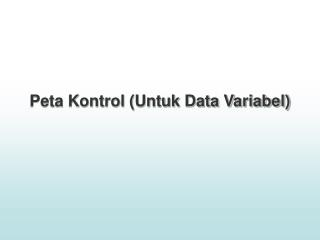Download PresentationPeta Kontrol (Untuk Data Variabel)

# Peta Kontrol (Untuk Data Variabel) - PowerPoint PPT PresentationDownload Presentation## Peta Kontrol (Untuk Data Variabel)

- - - - - - - - - - - - - - - - - - - - - - - - - - - E N D - - - - - - - - - - - - - - - - - - - - - - - - - - -
##### Presentation Transcript

1. Peta Kontrol (Untuk Data Variabel)

2. Peta Kontrol (Untuk Data Variabel)

3. Variabel dan Atribute • Pengertian Variabel • Pengertian Atribut • Perbedaan antara variabel dan atribut

4. Data Group • Tujuan analisa kemampuan proses • Sumber-sumber keuangan • Urut-urutan produksi • Identifikasi bagan kendali yang berasal dari sumber-sumber subgroup yang berbeda • Indeks kemampuan proses • Eksperimentasi

5. np-chart • The np Chart can be used for the special case when the subgroups are of equal size. Then it is not necessary to convert nonconforming counts into the proportions phat(i). Rather, one can directly plot the counts x(i) versus the subgroup number i.

6. Tahap-tahap Membuat np Chart • Determine the size of the subgroups needed. The size, n, has to be sufficiently large to have defects present in the subgroup most of the time. If we have some idea as to what the historical rate of nonconformance, p, is we can use the following formula to estimate the subgroup size: • n=3/p • Find find pbar.

7. Find the UCL and LCL where: • Plot the centerline pbar, the LCL and UCL, and the process nonconforming counts, the x(i)'s. • Interpret the control chart. Only if a point is outside the +/- 3 sigma range is the process considered to be out of control.